Array creation using Comprehensions and Generators in Julia

• Last Updated : 17 May, 2020

Julia is a language designed for high-level performance and can support interactive use as well. It has many descriptive datatypes and type-declarations can be used to solidify the programs. Julia is slowly climbing the ladder and gaining the interest of many Data Scientists and machine learning scientists today. It is comparatively faster than Python as it is designed to implement the math concepts like linear algebra and matrix representations. Excellent for numerical computation, this language has multiple dispatches for defining data types like numbers and arrays.

Arrays using Comprehensions

Julia arrays use square brackets([ ]) for list comprehensions just like Python or MATLAB. It consists of three kinds of arrays. Array comprehension is a very powerful way to construct an array. The resulting array depends on the type of data used while construction.
Syntax:

[expression for element = iterable]

For 1-D Arrays:

 # Julia array using for looptwice = []for i in 1:5    push!(twice, 2i)

can also be written and will give the same output as

 # Using Comprehensiontwice = [2x for x=1:5]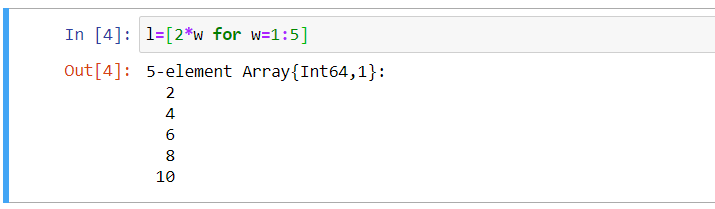For 2-D Arrays:

 # Creating 2D array using comprehension  u = [x + 2y for x in 1:5, y in 0:1]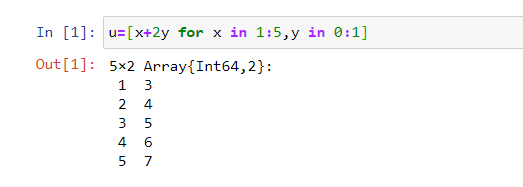For 3-D Arrays:

 # Creating 3D arrays using comprehension  p = [x + 2y + 3z for x in 1:4, y in 0:1, z in 1:3]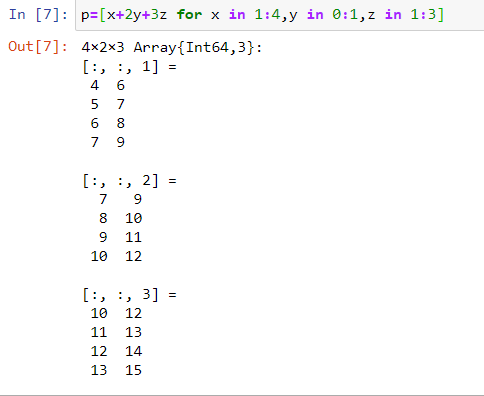Arrays using Generators

This does not exactly return an array type data structure but a generator type data structure. It uses parentheses ‘( )’ instead of the square brackets as in list comprehensions. The syntax else is very similar to that of the above.
The object can be iterated to produce values when needed instead of allocating an array and storing them in advance. The series of data below are not allocated any memory unlike the examples above.

Syntax:

(expression for element = iterable)

For 1-D Arrays:

 # Creating 1D Array using Generators  j = (2x for x = 1:5)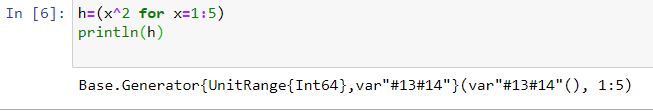For 2-D Arrays:

 # Creating 2D Arrays using Generatorsu = (x + 2y for x in 1:5, y in 0:1)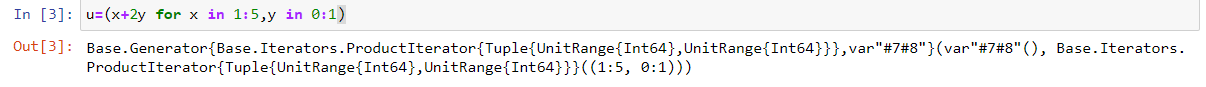For 3-D Arrays:

 # Creating 3D arrays using Generators  p = (x + 2y + 3z for x in 1:4, y in 0:1, z in 1:3)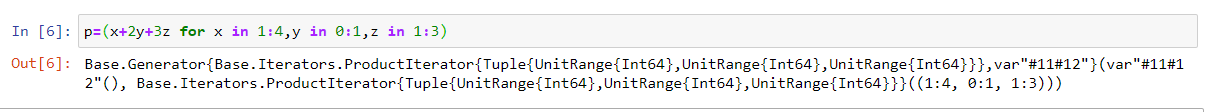My Personal Notes arrow_drop_up